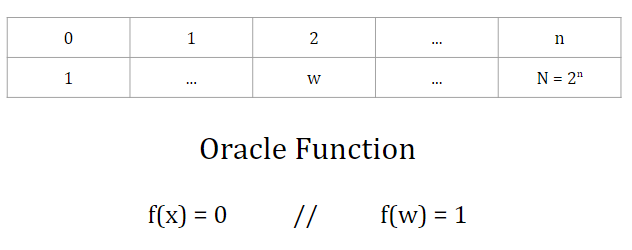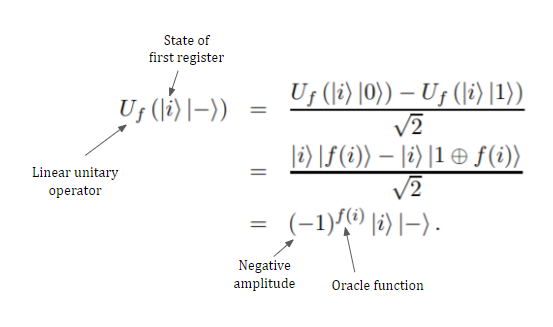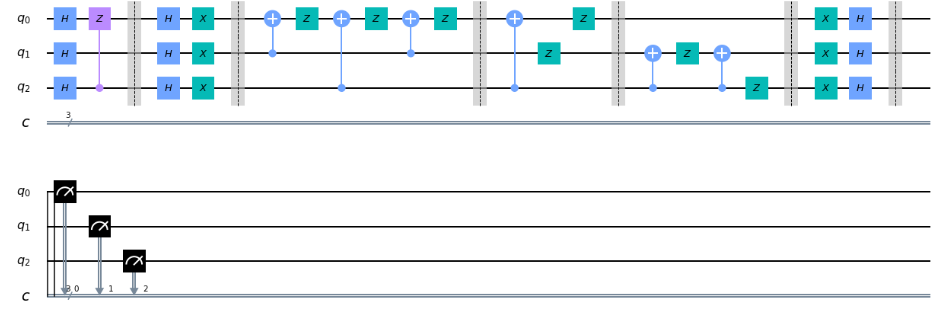# Grover’s Search Algorithm Using IBM’s Qiskit

Devised by Lov Grover in 1996, Grover’s Algorithm provides an incredible speedup from its classical counterpart.

It’s a searching algorithm that allows us to find a certain element in its respective list in O(√N) time, rather than O(N) time and thus, the quadratic speedup. N is the size of the database. While it’s not exponential, the speedup is still quite significant when N is large.

And it’s not just a regular searching algorithm either: it allows us to search unsorted databases without actually going each individual entry.

While it’s described as a searching problem, a more accurate description would be ‘inverting a function’. Given function y = f(x), the algorithm allows us to calculate x (the input in a database) when given y (output of database).

In some sense, if you had a cabinet full of empty drawers and one of those drawers had a book inside (and you were desperately looking for that book)… Grover’s algorithm would be able to find it for you!

# Grover’s Algorithm and Quantum Computing

Quick explanation of the math behind Grover’s algorithm and then implementation of the algorithm for 2, 3, and 4 qubits!

## In the context of quantum computers:

Going back to the analogy of a cabinet, think of the database as the cabinet, all the drawers as the elements in our database, and the book as element w.

Our problem: we need to find the index of the target state, w, among a list of N = 2^n elements where n = # of qubits and N = total size of the list.

• Quick caveat: this means we need to know the size of the database. Or in other words, we need to know the value of n to solve the problem. In real life, we would need to count the number of items in the list before applying the algorithm.The database where you’re looking for w and the oracle function that is being used

The solution: in simple terms, the Grover’s algorithm is made up of some oracle function (outputs 1 if w is found and 0 otherwise) that needs to run √N times in order to find w.

Of course, in reality, it’s a lot more complex than this… so here it is:

1. Put all the qubits into uniform /equal superposition: ensures all qubits have the same expectation value (same probability amplitude)

2. Apply the oracle reflection: mark the target element by negating its sign (you can think of it as if the operation flipped it)

It does something like this:This would be applied to all states (aka all the qubits in the circuit)

Don’t worry about the specifics — I just want you to look at the last step of the equation. The f(i) represents the oracle function. Similar to the image I showed you above, if i does not satisfy the condition (being the desired state), then the function is equal to 0. Something like this:

And so, if it’s our desired state, the negative sign stays in front of the state because (-1)¹ = -1. If it’s not our desired state, the state remains positive since f(i) = 0, which means that (-1)⁰ = 1. This effectively isolates or ‘marks’ the element we’re searching for with a negative amplitude.

The result is obtained by quantum parallelism (think: parallel universes, so you can do the same thing to all universes at the same time). In a sense, it’s possible to ‘see’ all the database elements simultaneously in order to perform the above function, which is what makes Grover’s algorithm so different from classical search algorithms.

3. Apply the Grover diffusion (an additional reflection): this operation amplifies the amplitude of the desired element

It’s also called the inversion about the mean because if we were to geometrically represent it, we would be ‘inverting’ the vector representing the qubit over its mean (but geometry = too much math, so we’ll ignore this for now, but it’s useful to know its other names).

Ok, that was a lot of math, so here’s a more visual representation:

## Here’s how to implement this algorithm using IBM’s Qiskit platform:

You can find the full tutorial with annotated code and useful visualizations here on my github. I won’t go through any code here but instead, I’ll show you the visualizations of each circuit and the results of running the circuit.

Grover’s algorithm for 2 qubits: oracle for state |11⟩

The simulator results tell us that there’s a 100% chance the found element is the desired element (in other words, the resulting amplified amplitude = 1 so the algorithm is 100% certain about the result). The computation results, performed on an actual quantum computer tell us that the algorithm is 92.6% certain about the results.

As we scale in the number of qubits, you’ll notice that the quantum computation completely loses accuracy. This is due to quantum error.

Grover’s algorithm for 3 qubits: oracle for state |111⟩Note: the circuit continues on the second line because it can’t fit in one line.

As you can see, as we scale the problem, the circuit almost gets exponentially more complex. This is because some of the circuits that we are using for lesser qubits cannot be used for more qubits (meaning we need to entirely recreate the circuit, from scratch).

Grover’s algorithm for 4qubits: oracle for state |1111⟩

And that’s all. Make sure to check out my github page (shameless self promo hahaha) to see how we can construct these circuits from scratch!

## So, how realistic is all of this?

The short answer is not really.

The long answer is our quantum hardware hasn’t caught up to the software and many quantum algorithms are suitable for a specific subset of problems.

It’s quite difficult to prove quantum advantage, never mind quantum supremacy, for algorithms like Grover’s because they are classical problems that have been developed alongside the best of conventional algorithms.

Of course, it’s not fair to compare quantum algorithms that are still in their infancy with mature classical algorithms that have been developed for decades, but we should always question practical significance and relevance.

Here’s when Grover’s algorithm would actually be useful / practical:

1. A search application where classical methods don’t provide sufficient scalability: meaning if we computed classically the problem wouldn’t be solvable when the database gets really big.
2. An implementation of Grover’s search with an asymptotic worst-case runtime (the time needed to find the element): meaning the runtime needs to be less than that of any classical algorithm (spoiler alert, there hasn’t been one yet).
3. A Grover’s search with an practical runtime for search applications: meaning even with a large database, the algorithm can still compute within a reasonable amount of time (and not take thousands of years).

But you see, the problem is the real-life database applications rarely meet all of these requirements, nevermind just one. And, we would also need to convert the classical problem into a quantum one (aka map it onto the qubits).

So it isn’t likely that quantum computers will crack all encryption methods soon (but it is likely inevitable).

In the meantime, there has been a significant amount of promising research in regards to implementing Grover’s algorithm to solve NP-complete problems (so yes, don’t lose hope!)

A NP (non-deterministic polynomial) complete problem is a set of all the division problems (questions w/yes or no answers) wherein the ‘yes’ answer can be verified in polynomial time.

Some cool applications of Grover’s Algorithm:

• Travelling salesman problem: (it is a graph traversal problem) given a list of cities connected by roads and a gasoline budget of k dollars, a route must be determined so that a salesman visits each city only once, returns to the starting city, and spends k or fewer dollars on gasoline.
• Graph 3-coloring: (visualize a graph with 3 different colors and an arbitrary number of vertices connected by edges) the goal is to find an assignment of colors to all vertices, if one exists, so that no two vertices connected by an edge have the same color.
• Boolean 3-satisfiability (3-SAT): This problem requires finding an assignment of true/false values to variables of a Boolean function f in conjunctive normal form (CNF) such that f is true. In CNF, the function consists of the conjunction (logical AND) of clauses, and each clause is the disjunction (logical OR) of Boolean variables in complemented or uncomplemented form. In 3-SAT, each clause contains three variables, though the number of distinct variables and clauses might vary.

So, as you can see, the ‘unstructured database problem’ is a really diverse range of problems that have an incredible amount of applications in the real world as purely theoretical as these NP-complete problems may seem.

If you’re interested in learning more, definitely checkout Qiskit’s collection of community tutorials on optimization problems (many of which apply Grover’s algorithm for these NP-complete problems):

The possibilities of implementations of quantum computing are endless, even though the development of the applications of algorithms are still in their infancy (we’re getting there)!

So, try it yourself!

--

--